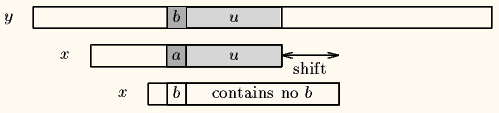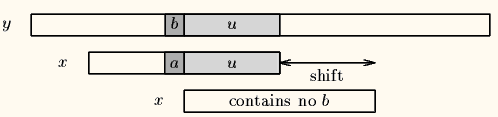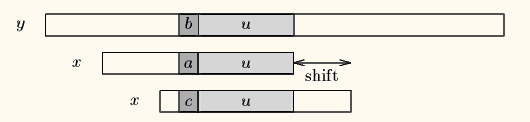# 字符串匹配算法

4 篇文章 0 订阅

## 朴素的匹配算法

- 如果达到子字符串末尾，就返回母串中子串首字符对应位置
- 如果遇到不匹配字符就回退到子串首字符对应母串的位置的下一个字符进行比较

public class Solution {
public int strStr(String haystack, String needle) {
if(needle==null || needle.length()==0){
return 0;
}
int p =0;
for(int i=0; i<haystack.length(); i++){
if(haystack.charAt(i)==needle.charAt(p)){
p++;
}else{
i-=p;
p =0;
}
if(p==needle.length()){
return i-p+1;
}
}
return -1;
}
}

## KMP算法

KMP算法的核心是一个字串回退数组next,来确定当字符不匹配时字串的回退位置，这个数组只与字串特征有关，表示前缀与后缀的最大匹配长度。

- 如果已匹配部分后缀与前缀匹配长度为零，那就只能直接跳过已匹配部分
- 如果已匹配部分后缀与前缀有匹配部分，那么就将字串指针跳到字串已匹配部分前缀与后缀最大匹配长度位置
- 如果下一个字符还是不匹配，重复上面的跳转，直到p为零


- 首先将另一字串置于字串最大匹配部分
- 如果新的字符不匹配，就找已匹配部分的最大匹配长度，然后对比新字符，就像KMP算法匹配字符串

public class Solution {
public static void main(String[] args) {
String haystack = "hello, how are you";
String needle = "how";
System.out.println(strStr(haystack,needle));
}

public static int strStr(String haystack, String needle){
int[] next = getNext(needle);
for(int i=0,j=0; i<haystack.length(); i++){
while(j>0 && haystack.charAt(i)!=needle.charAt(j)){
j=next[j-1];
}
if(haystack.charAt(i)==needle.charAt(j)){
j++;
}
if(j==needle.length()){
return i-j+1;
}
}
return -1;
}
public static int[] getNext(String needle){
int[] next = new int[needle.length()];
next = 0;
for(int i=1,j=0; i<next.length; i++){
while(j>0 && needle.charAt(i)!=needle.charAt(j)){
j = next[j-1];
}
if(needle.charAt(i)==needle.charAt(j)){
j++;
}
next[i] = j;
}
return next;
}
}  

## Rabin_Karp算法

• 该算法核心是将字符串的比较转化为其对应的Hash值的比较，关键在于如何根据母串中P[s,s+m]的Hash值在O（1）的时间内计算出P[s+1,s+1+m]的Hash值。
• 算法复杂度最坏为O((n-m+1)*m)，出现在Hash碰撞比较多的时候， 但是平均复杂度为O(n)
• 如下为一个简单的数字匹配算法，关键算法hash = ((hash-(source.charAt(i)-'0')*h) * 10 + source.charAt(i+m) - '0')%q
public class Demo {
public static void main(String[] args) {
String haystack = "1012132343";
String needle = "12";
System.out.println(indexOfRK(needle, haystack));
}
public static int indexOfRK(String sub, String source) {
int m = sub.length();
int n = source.length();

long h = 1;
long q = 127;
for(int i=0; i<m-1; i++){
h=h*10%q;
}

long subHash = 0;
long hash = 0;

for (int i = 0; i < m; i++) {
subHash = (subHash * 10 + sub.charAt(i) - '0')%q;
hash = (hash * 10 + source.charAt(i) - '0')%q;
}
for (int i = 0; i <= n - m; i++) {
if (subHash == hash && check(sub, source, i)) {
return i;
}
if (i < n - m) {
hash = ((hash-(source.charAt(i)-'0')*h) * 10 + source.charAt(i+m) - '0')%q;
if(hash<0){
hash+=q;
}
}
}
return -1;
}

private static boolean check(String sub, String source, int index) {
for (int i = index, j = 0; j < sub.length(); i++, j++) {
if (source.charAt(i) != sub.charAt(j)) {
return false;
}
}
return true;
}
}  

## BM算法

（1）坏字符算法

Case1：模式串中有对应的坏字符时，让模式串中最靠右的对应字符与坏字符相对（PS：BM不可能走回头路，因为若是回头路，则移动距离就是负数了，肯定不是最大移动步数了），如下图。Case2：模式串中不存在坏字符，很好，直接右移整个模式串长度这么大步数，如下图。（2）好后缀算法

Case1：模式串中有子串和好后缀完全匹配，则将最靠右的那个子串移动到好后缀的位置继续进行匹配。Case2：如果不存在和好后缀完全匹配的子串，则在好后缀中找到具有如下特征的最长子串,使得P[m-s…m]=P[0…s]。Case3：如果完全不存在和好后缀匹配的子串，则右移整个模式串。

（3）移动规则

BM算法的移动规则是：

BM算法是每次向右移动模式串的距离是，按照好后缀算法和坏字符算法计算得到的最大值。

shift（好后缀）和shift（坏字符）通过模式串的预处理数组的简单计算得到。坏字符算法的预处理数组是bmBc[]，好后缀算法的预处理数组是bmGs[]。

12-041万+
05-2511912-056009
06-15341
05-077万+
06-137611
09-201152
10-252万+
01-2031
11-243959
01-07720
06-252万+
04-24617
06-272809
01-2922
10-114146
11-203610
05-128552点击重新获取扫码支付余额充值